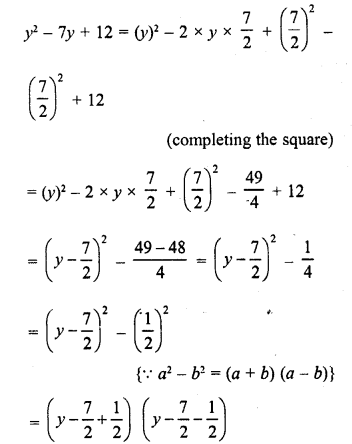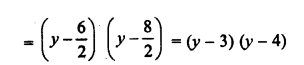# RD Sharma Class 8 Solutions Chapter 7 Factorizations Ex 7.9

In this chapter, we provide RD Sharma Class 8 Solutions Chapter 7 Factorization Ex 7.9 for English medium students, Which will very helpful for every student in their exams. Students can download the latest RD Sharma Class 8 Solutions Chapter 7 Factorization Ex 7.9 Maths pdf, free RD Sharma Class 8 Solutions Chapter 7 Factorization Ex 7.9 Maths book pdf download. Now you will get step by step solution to each question.

### RD Sharma Solutions for Class 8 Chapter 7 Factorization Ex 7.9Download PDF

Factorize each of the following quadratic polynomials by using the method of completing the square.
Question 1.
p2 + 6p + 8
Solution:
p2 + 6p + 8
= p2 + 2 x p x 3 + 32 – 32 + 8   (completing the square)
= (p2 + 6p + 32) – 1
= (p + 3)2 – 12
= (P + 3)2 – (1)2       { ∵ a2 + b2  = (a+b) (a-b)}= (p +3+1) (p + 3 -1)
= (p+4) (p+ 2)

Question 2.
q2 – 10q + 21
Solution:
q2 – 10q + 21
= (q)2 – 2 x q x 5 + (5)2 – (5)2 + 21   (completing the square)
= (q)2 – 2 x q x 5 + (5)2 -25+21
= (q)2-2 x q x 5 + (5)2 – 25 +21
= (q)2-2 x q x 5 + (5)2 – 4
= (q – 5)2 – (2)     {∵a2 – b2 = (a + b) (a – b)}
= (q- 5 + 2) (q-5-2)
=(q- 3) (q-7)

Question 3.
4y2 + 12y + 5
Solution:
4y+12y + 5
= (2y)2 + 2 x 2y x 3 + (3)2 – (3)2 + 5    (completing the square)
= (2y + 3)2 – 9 + 5
= (2y + 3)2 – 4
= (2y + 3)2-(2)2   {∵ a2 – b2 = (a + b) (a – b)}
= (2y + 3 + 2) (2y + 3 – 2)
= (2y + 5) (2y+ 1)

Question 4.
p2 + 6p-
16
Solution:
p2 + 6p – 16
= (p)2 + 2 x  p x 3 + (3)2 – (3)2 – 16    (completing the square)
= (p)2 + 2 x p x 3 + (3)2 – 9 – 16
= (p + 3)2 – 25
= (p + 3)2 – (5)2     {∵ a2 -b2 = {a + b) (a – b)}
= (p + 3 + 5)(p + 3-5)
= (p + 8) (p – 2)

Question 5.
x2 + 12x + 20
Solution:
x2 + 12x + 20
= (x)2 + 2 x x x 6 + (6)2 – (6)2 + 20   (completing the square)
= (x)2 + 2 x x x6 + (6)2 -36 + 20
= (x + 6)2 -16
= (x + 6)2 – (4)2   {∵ a2 – b2 = (a + b) (a – b)}
= (x + 6 + 4) (x + 6 – 4)
= (x + 10) (x + 2)

Question 6.
a2 – 14a – 51

Solution:
a2 – 14a-51
= (a)2 – 2 x x 7 + (7)2 – (7)2 – 51       (completing the square)
= (a)2 – 2 x a x 7 + (7)2 – 49 – 51
= (a – 7)2 – 100
= (a – 7)2 – (10)2    {∵  a2 – b2 = (a + b) (a – b)}
= (a – 7 + 10) (a – 7 – 10)
= (a + 3) (a – 17)

Question 7.
a2 + 2a – 3
Solution:
a2 + 2a – 3
= (a)2 + 2 x a x 1 + (1)2 – (1)2 – 3   (completing the square)
= (a)2 + 2 x a x 1 + (1)2 – 1 – 3
= (a + 1)2 – 4
= (a + 1)2 – (2){∵ a2 – b2 = (a + b) (a – b)}
= (a + 1 + 2) (a + 1 – 2)
= (a + 3) (a – 1)

Question 8.
4x2 – 12x + 5
Solution:
4x2 – 12x + 5
= (2x)2 – 2 x 2x x 3 + (3)2 – (3)2 + 5  (completing the square)
= (2x)2 – 2 x 2x x 3 + (3)2 -9 + 5
= (2x – 3)2 – 4
= (2x – 3)2 – (2)2      {∵ a2 – b2 = (a + b) (a – b)}
= (2x – 3 + 2) (2x – 3 – 2)
= (2x – 1) (2x – 5)

Question 9.
y2 – 7y + 12
Solution:Question 10.
z2-4z-12

Solution:
z2 – 4z – 12
= (z)2 – 2 x z x 2 + (2)2 – (2)2 – 12  (completing the square)
= (z)2 – 2 x z x 2 + (2)2 – 4 – 12
= (z-2)2-16
= (z-2)2-(4)2   {∵ a2 – b2 = (a + b) (a – b)}
= (z – 2 + 4) (z – 2 – 4)
= (z + 2)(z-6)

All Chapter RD Sharma Solutions For Class 8 Maths

—————————————————————————–

All Subject NCERT Exemplar Problems Solutions For Class 8

All Subject NCERT Solutions For Class 8

*************************************************

I think you got complete solutions for this chapter. If You have any queries regarding this chapter, please comment on the below section our subject teacher will answer you. We tried our best to give complete solutions so you got good marks in your exam.

If these solutions have helped you, you can also share rdsharmasolutions.in to your friends.